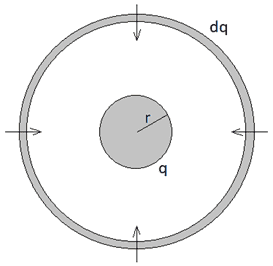# Electrostatic Potential Energy of a Sphere/Shell of Charge

sophiatev
Homework Statement:
Find the electrostatic potential energy of a sphere of uniform charge density, and that of a shell of uniform surface charge density.
Relevant Equations:
We are only meant to use expressions for potential energy (i.e. we know that outside the sphere/shell, the potential has the form q/(4 * pi * epsilon * r)). We are not meant to use expressions for electrostatic potential energy of the form W = 1/2 * (integral of charge density * V) or W = epsilon/ 2 * (integral of the magnitude of the electric field^2). (Sorry, I tried to Latex these equations but I couldn't for some reason).
So it seems the typical way to approach this problem is to consider the sphere when it has charge q and radius r. With uniform charge density ##\rho##, this becomes ##q = 4/3 \pi r^3 \rho## and so ##dq = 4 \pi r^2 dr \rho##. Using our expression for the potential outside of the sphere, we find that the work necessary to bring this charge dq in from infinity is ## qdq / 4 \pi \epsilon_0 = 4 \pi r^4 \rho^2 dr' / 3 \epsilon_0##. We integrate this expression from 0 to the final radius of the sphere and get that ## W = 3Q^2 / 20 \pi \epsilon_0 a##, where Q is the total charge of the sphere and a the radius. But when I try to imagine what this means physically, it is not at all obvious to me that this is the right expression. We bring the charge dq in from infinity, but then we also have to effectively "spread" this charge out over the sphere once it arrives. Why that happens "naturally", without any additional work, is not at all obvious to me. I also thought of bringing in a thin spherical shell of radius r and charge ##dq = 4 \pi r^2 dr \rho## from infinity, but then we also have to take into account the work done in assembling that shell at infinity.

The case with finding the electrostatic potential energy of a shell of uniform surface charge density is even less obvious to me. It seems the general prescription in this case is even more hand-wavy - you assume you already have a shell of charge q assembled, and so the work necessary to add a charge dq to it is simply ##qdq / 4 \pi \epsilon_0 a##, where a is the radius of the shell. Integrating this from 0 to Q we find that the final energy is ##W = Q^2 / 8 \pi \epsilon_0 a##. But this seems to completely neglect how the "initial shell" formed that we build upon by adding charge. In the case of the sphere we start with a point charge, then add an infinitely thin shell around it, then another one, etc. until we build up the sphere. But it seems in the case of the shell we have to start with a shell of some charge, and "neglect" the work necessary in assembling that initial shell.

Homework Helper
2022 Award
Homework Statement:: Find the electrostatic potential energy of a sphere of uniform charge density, and that of a shell of uniform surface charge density.
Relevant Equations:: We are only meant to use expressions for potential energy (i.e. we know that outside the sphere/shell, the potential has the form q/(4 * pi * epsilon * r)). We are not meant to use expressions for electrostatic potential energy of the form W = 1/2 * (integral of charge density * V) or W = epsilon/ 2 * (integral of the magnitude of the electric field^2). (Sorry, I tried to Latex these equations but I couldn't for some reason).

The case with finding the electrostatic potential energy of a shell of uniform surface charge density is even less obvious to me.

I think the question is inherently related to the self-energy of a point charge and is not at all elementary. In fact it is better to think about assembling the spherical shell from infinity in a spherically symmetric manner. Then we ask the additional work required to bring that shell to some finite radius r. One might think this is cheating but it all works out.
But a finite "point" charge is a singularity and classically requires infinite forces to contain it. get used to not worrying about it and in fact we seldom need to.

My LaTeX seems kaput also

Homework Helper
Gold Member
When “squeezing” a shell of charge ##dq## from infinity onto a ball of charge ##q## of radius ##r##, the work you do consists of the work ##dW_1## done against the interaction between ##dq## and ##q## plus the work ##dW_2## done against the interaction between elements of charge within the shell with other elements of charge within the shell. On dimensional grounds, ##dW_1 \sim qdq/(\epsilon_0 r)## and ##dW_2 \sim (dq)^2/(\epsilon_0 r)##. So ##dW_2## is of second order in ##dq## and can be neglected.